# imoverlay and imagesc

In March 2006 I wrote about how to overlay a specified color onto specified pixels of a grayscale or RGB image. I put a utility function (imoverlay) for performing this operation on the MATLAB Central File Exchange.

Earlier this week, Matt W. posted a review of that submission, suggesting that additional documentation might be helpful for people displaying image data that's not in the range [0, 1]. MATLAB users often call imagesc to "autoscale" image data, and Image Processing Toolbox users can call imshow(I,[]) to get a similar effect.

Here's an example using imagesc.

L = membrane(1, 100);
imagesc(L), axis equal, colormap(hot)
axis equal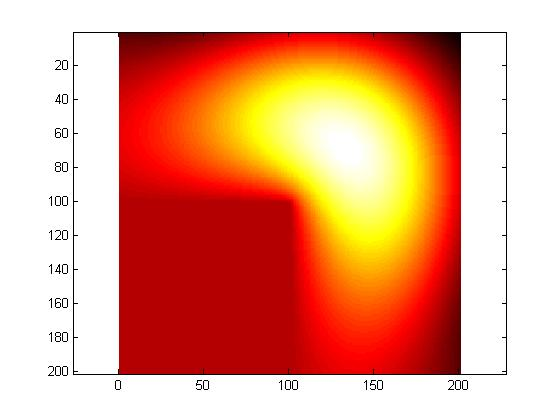Note that L has negative values.

min(L(:))
ans =

-0.3338


max(L(:))
ans =

1



imagesc automatically scales the values of L "into" the colormap, so that the minimum value is displaying using the first colormap color and the maximum value is displayed using the last colormap color. (See my post about scaled indexed images.)

Although Matt's specific suggestion was about getting imoverlay to work with this data, there's a more general concept here about how to save images like the one above to image file formats, such as JPEG or PNG. Image file formats have generally much less flexibility than MATLAB about displaying pixel values as colors. In particular, the most common formats don't have the notion of scaling an arbitrary range of data values into a colormap. In addition, the JPEG format doesn't even have the notion of using a colormap at all.

We could try writing the L values directly into a JPEG file, like this:

imwrite(L,'membrane1.jpg')
imshow membrane1.jpg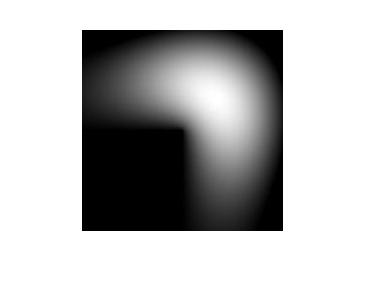But we lost all the color information, and there's no automatic data scaling. All the values of L less than 0 were simply clipped to black in the JPEG file.

So how can we turn L and the hot colormap into something that can be stored in a JPEG file?

We can do it in three steps:

• Scale L into the dynamic range [0, 1] by using mat2gray.
• Convert the result into a normal (unscaled) indexed image using gray2ind.
• Convert the indexed image into RGB form by using ind2rgb.
gray = mat2gray(L);
X = gray2ind(gray, 256);
rgb = ind2rgb(X, hot(256));

imwrite(rgb, 'membrane2.jpg')
imshow membrane2.jpg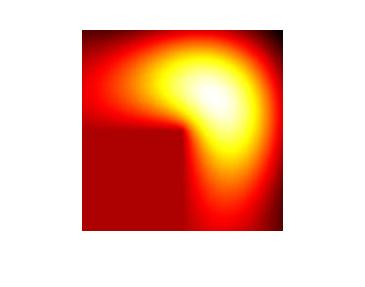Now that the membrane image is in RGB form, we can use imoverlay. For example, let's overlay all the negative pixels with a light blue color.

mask = L < 0;
light_blue = [.6 .6 1];

imwrite(overlay, 'membrane3.jpg')
imshow membrane3.jpg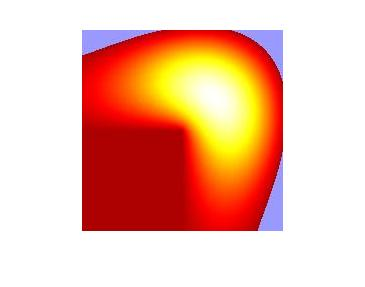Of course, every time you launch MATLAB you see this membrane shape in a more familiar form that looks something like this:

membrane(1,50)
colormap(hot)
camlight
shading interp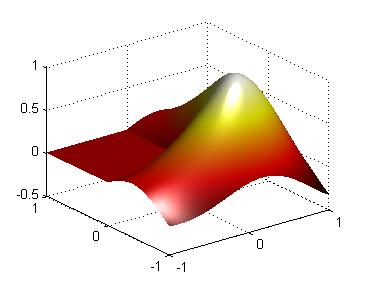The L-shaped membrane has played a fascinating role in the history of MATLAB and The MathWorks. If you are interested in the origins and history of MATLAB, you should check out the Origins of MATLAB video by MATLAB creator and company co-founder Cleve Moler.

Published with MATLAB® 7.4

|